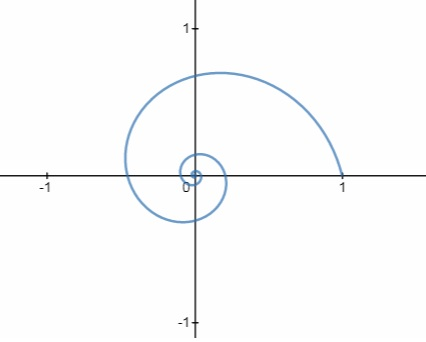# r = e^{\frac{-\theta}{4}} How would you go about graphing this polar equation on the polar plane?

## Question:

{eq}r = e^{\frac{-\theta}{4}} {/eq}

How would you go about graphing this polar equation on the polar plane?

## Graphs:

The graphical method of the polar curve equation plotting is done with the graphical calculator and the polar curve that is plotted will be in polar coordinates. With that use, we plot the points too on the curve.

## Answer and Explanation:

The polar equation that is given is:

{eq}r = e^{\frac{-\theta}{4}} {/eq}

Now we plot on the polar curve as follows:#### Learn more about this topic:Graphs: Types, Examples & Functions

from High School Algebra II: Help and Review

Chapter 16 / Lesson 11
355K